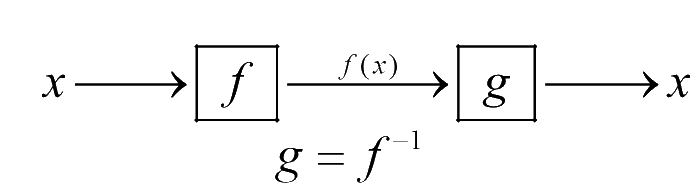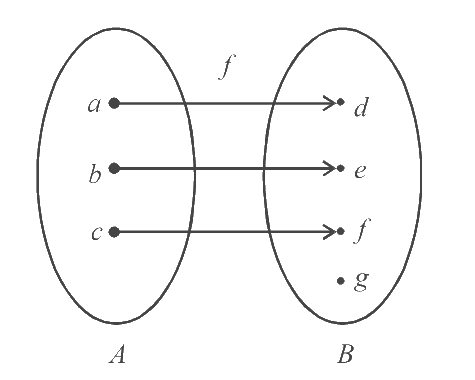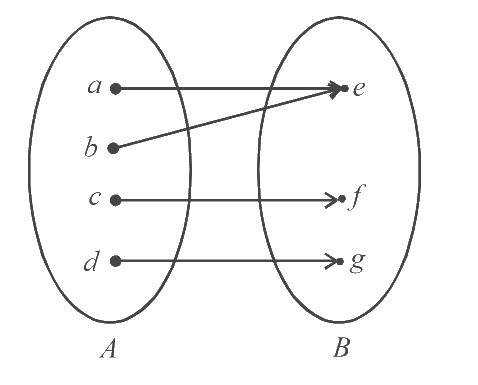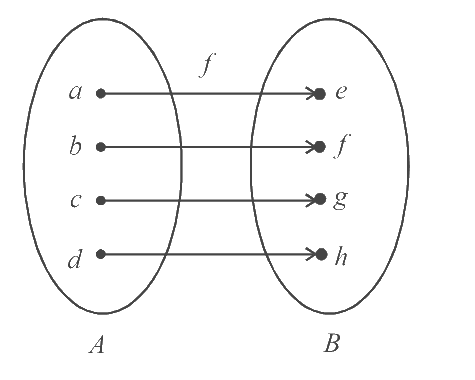# Inverse of a Function

The inverse of a function $$f(x)$$ is a function $$g(x)$$ such that if $$f$$ maps an element $$'a'$$ to an element $$'b',$$ $$g$$ maps $$'b'$$ to $$'a'.$$

In more formal terms, $$g\left( {f\left( x \right)} \right) = x.$$ That is, $$g$$ reverses the action of $$f$$ on $$x.$$An inverse cannot exist for every function. To see why, consider  $$f:\,\,A \to B$$  where $$\text{A}$$ is the domain and $$\text{B}$$ the co-domain. Consider the following maps:

(a)  This is an into map (into function). If we take the ‘inverse map’ (from $$\text{B}$$ to $$\text{A,}$$ using the same ‘links’ as in $$f\,$$), we see that the element $$'g'$$ cannot be assigned to any element in $$\text{A.}$$ In other words, for an into function, some values in the co-domain are ‘left out’, and their ‘inverses’ do not exist.$$\Rightarrow$$               We cannot defined an inverse for an into function.

(b)  This is a many-one map (a many-one function).

If we take the inverse map, the element $$'e'$$ will be assigned to two elements in $$\text{A,}$$ that is, $$'a'$$ and $$'b'.$$

Hence, the inverse map cannot be a function.$$\Rightarrow$$               We cannot defined an inverse for a many-one function.

From this discussion, we conclude that for a function to be invertible, it should be one-one and onto (also called a bijective function).As is intuitively clear, we can easily define an inverse for the map above.

$\left( {{f^{ - 1}}(e) = a,\,\,{f^{ - 1}}(f) = b,\,\,{f^{ - 1}}(g) = c,\,\,{f^{ - 1}}(h) = d} \right).$